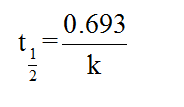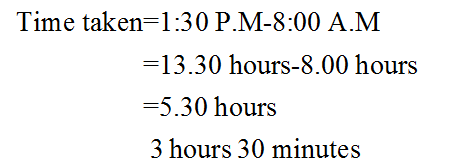# If 200. mg of fluorine-18 is shipped at 8:00 A.M., how many milligrams of the radioisotope are still active when the sample arrives at the radiology laboratory at 1:30 P.M.?Express the mass to three significant figures and include the appropriate units.

Question
14 views
If 200. mg of fluorine-18 is shipped at 8:00 A.M., how many milligrams of the radioisotope are still active when the sample arrives at the radiology laboratory at 1:30 P.M.?
Express the mass to three significant figures and include the appropriate units.
check_circle

Step 1

The term radioactive substances can be defined as the atoms which have the tendency to decay naturally. They can emit alpha particles, beta particles and gamma radiations as well.

Step 2

The half-life of a given radioactive substance can be termed as the time taken by the given amount of substance to get reduced to half as a result of the decay and thus, the emission of radiations.

The half -life of a given substance can be calculated from the given value of the rate constant by using the following equation as shown below:Step 3

The half-life of Fluorine-18 is 109.7 minutes.

The total time taken in the given shipment can be calculated as follows:...

### Want to see the full answer?

See Solution

#### Want to see this answer and more?

Solutions are written by subject experts who are available 24/7. Questions are typically answered within 1 hour.*

See Solution
*Response times may vary by subject and question.
Tagged in

### Chemistry